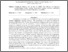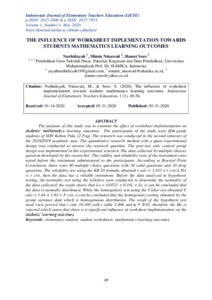# THE INFLUENCE OF WORKSHEET IMPLEMENTATION TOWARDS STUDENTS MATHEMATICS LEARNING OUTCOMES

Nur, HidayahdanMimin, NinawatidanSlamet Soro, SS (2020) THE INFLUENCE OF WORKSHEET IMPLEMENTATION TOWARDS STUDENTS MATHEMATICS LEARNING OUTCOMES. Indonesian Journal of Elementary Teachers Education, 1 (1). pp. 49-56. ISSN P-ISSN: 2615-2606 and E-ISSN: 2615-7853Preview
Text
THE INFLUENCE OF WORKSHEET IMPLEMENTATION TOWARDS STUDENTS MATHEMATICS LEARNING OUTCOMES.pdf

Download (458kB) | Preview

## Abstract

The purpose of this study was to examine the effect of worksheet implementation on students mathematics learning outcomes. The participants of the study were fifth-grade students of SDN Kebon Pala 12 Pagi. The research was conducted in the second semester of the 2018/2019 academic year. The quantitative research method with a quasi-experimental design was conducted to answer the research question. The post-test only control group design was implemented in this experimental research. The data collected by multiple choices question developed by the researcher. The validity and reliability tests of the instrument were tested before the instrument administered to the participants. According to Biserial Point Correlation, there were 40 multiple choice questions with 30 valid questions and 10 drop questions. The reliability test using the KR-20 formula obtained r calc = 1.033 > r crit 0.361 = r crit, then the data has a reliable instrument. Before the data analyzed in hypothesis testing, the normality test using the Liliefors were conducted to determine the normality of the data collected, the result shows that Lo = 0.0521 < 0.161 = Lt, it can be concluded that the data is normally distributed. While the homogeneity test using the Fisher test obtained F calc = 1.44 < 1.93 = F crit, it can be concluded that the homogeneity testing obtained by the group variance data which is homogeneous distribution. The result of the hypothesis test used t-test proved that t calc 10.169 with t table 2.006 and α = 0.05, therefore the Ho is rejected which states that there is a significant influence of worksheet implementation on the students learning outcomes.

Item Type: Article Fakultas Keguruan dan Ilmu Pendidikan > Pendidikan Guru Sekolah Dasar Mimin Ninawati 31 Aug 2020 07:33 31 Aug 2020 07:33 http://repository.uhamka.ac.id/id/eprint/4059

### Actions (login required)View Item